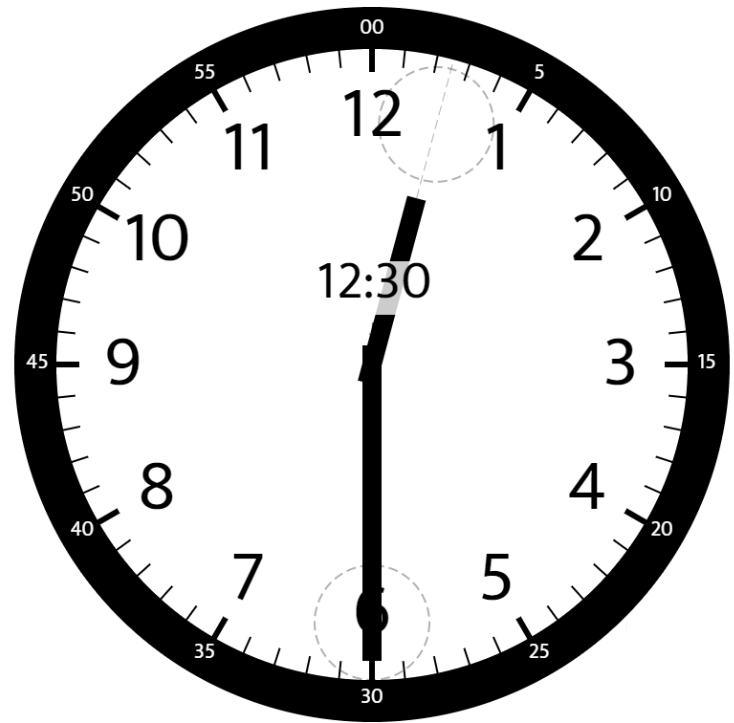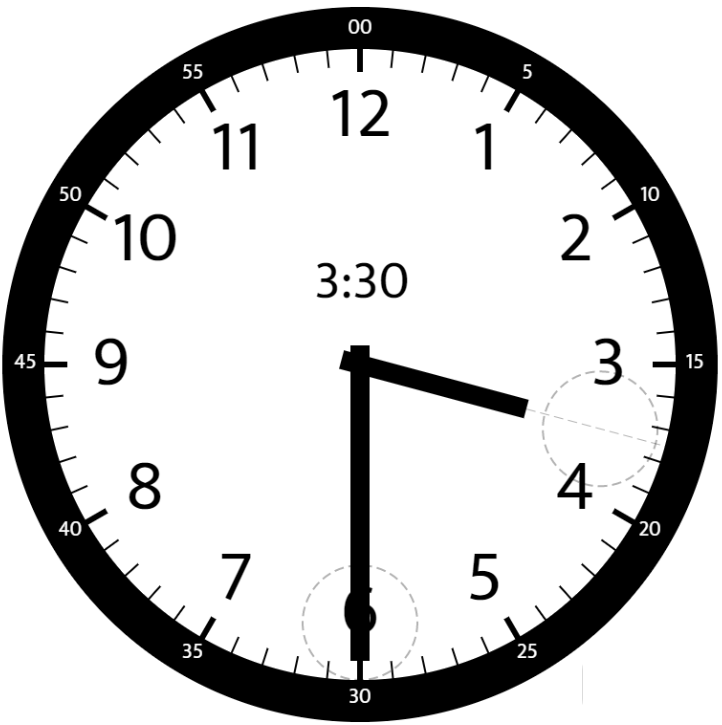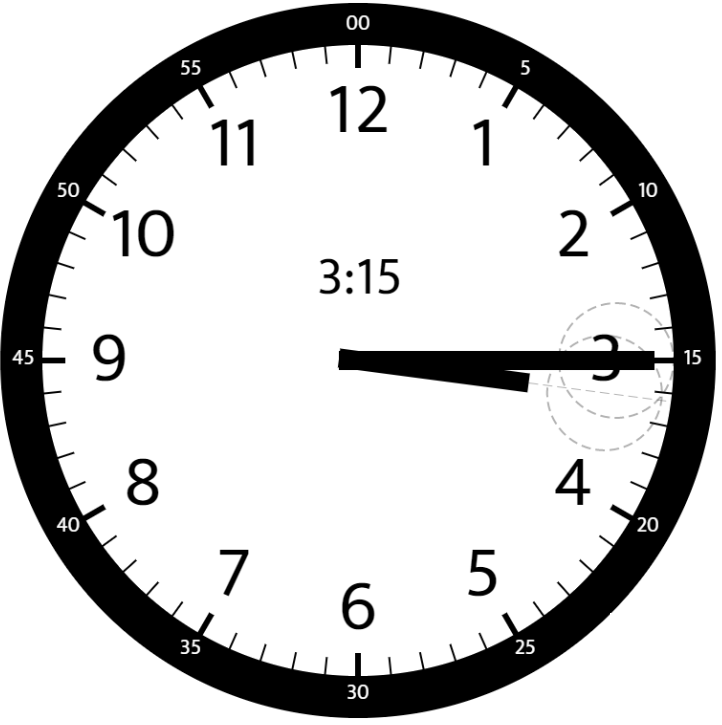## LeetCode-Python-1344. 时钟指针的夹角（数学）``````输入：hour = 12, minutes = 30

````````````输入：hour = 3, minutes = 30

````````````输入：hour = 3, minutes = 15

``````

``````输入：hour = 4, minutes = 50

``````

``````输入：hour = 12, minutes = 0

``````

• `1 <= hour <= 12`
• `0 <= minutes <= 59`
• 与标准答案误差在 `10^-5` 以内的结果都被视为正确结果。

emmm，还是水题，把时针相对12点的角度计算一下，再把分针相对12点的角度计算一下，

``````class Solution(object):
def angleClock(self, hour, minutes):
"""
:type hour: int
:type minutes: int
:rtype: float
"""
min_angle = minutes * 1.0 / 60 * 360
hour_angle = hour * 1.0 / 12 * 360 + minutes * 1.0 / 60 * 30

res = abs(hour_angle - min_angle)

return res if res < 180 else 360 - res``````